+关注继续查看

# 二、数学符号

• 红色框中的为输入、输出值。可以看到人名输出用1表示，反之用0表示；
• 绿色框中的$$x^{<t>},y^{<t>}$$表示对应红色框中的输入输出值的数学表示，注意从1开始。
• 灰色框中的$$T_x,T_y$$分别表示输入输出序列的长度，在该例中，$$T_x=9,T_y=9$$
• 黄色框中$$X^{(i)<t>}$$上的表示第i个输入样本的第t个输入值，$$T_x^{(i)}$$则表示第i个输入样本的长度。输出y也同理。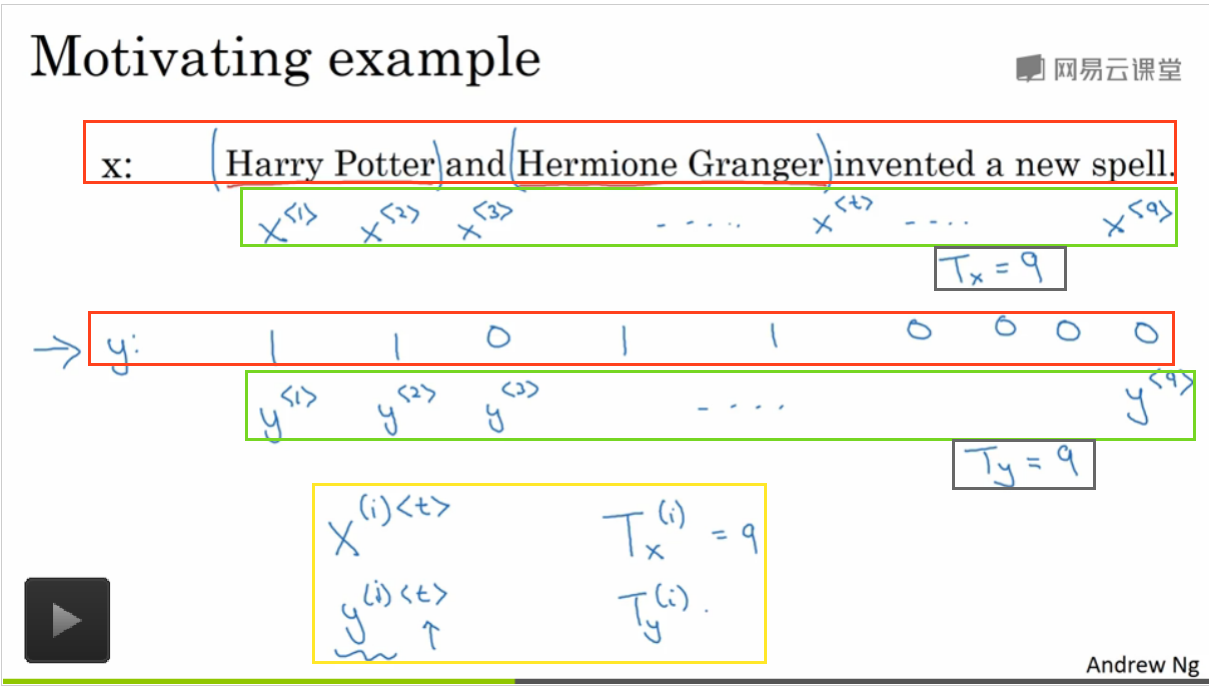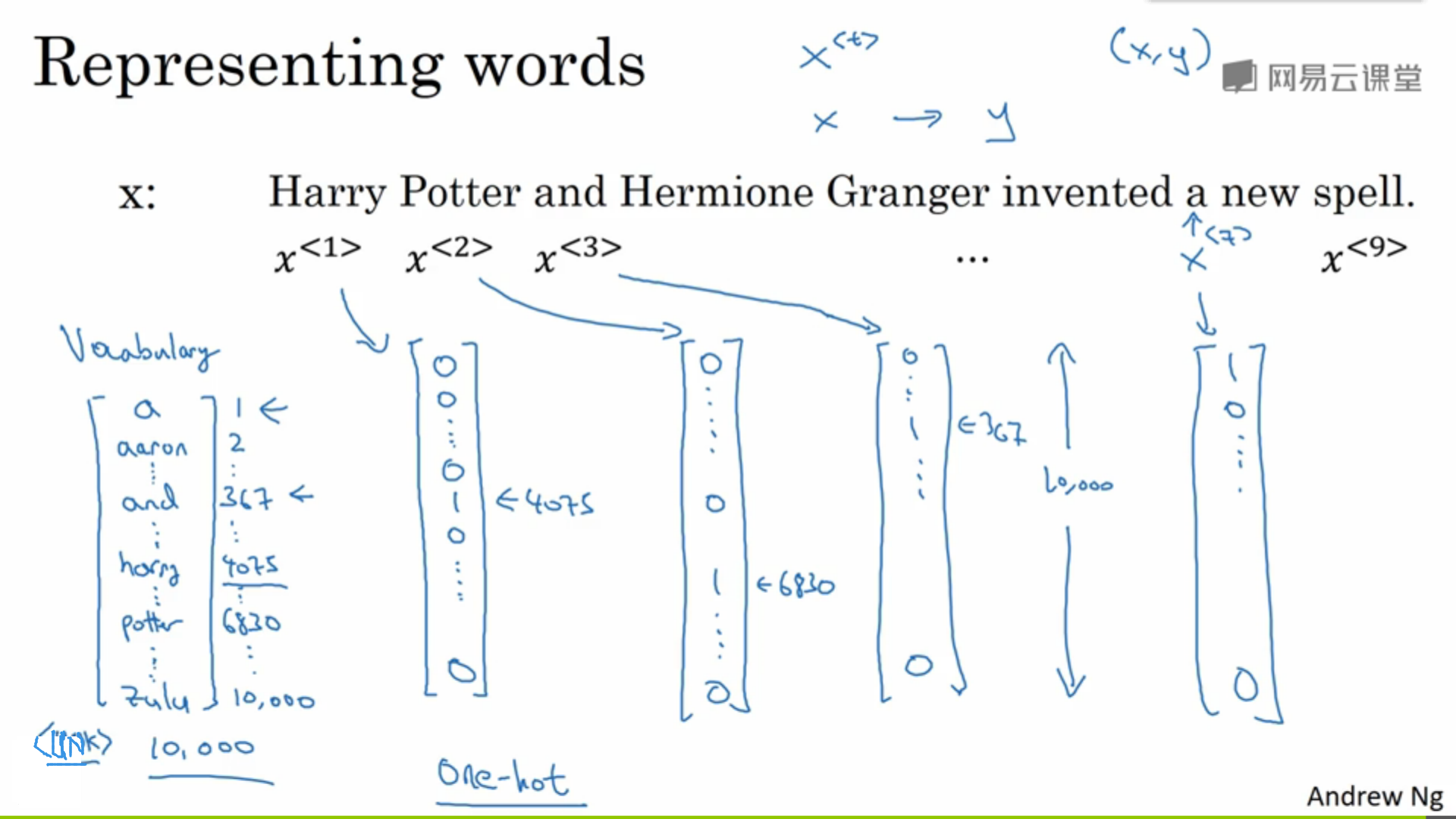# 三、循环神经网络模型

## 1.为什么不用标准网络

• 输入和输出的长度不尽相同
• 无法共享从其他位置学来的特征。例如上一节中的Harry这个词是用$$x^{<1>}$$表示的，网络从该位置学习了它是一个人名。但是我们希望无论Harry在哪个位置出现网络都能识别出这是一个人名的一部分，而标准网络无法做到这一点。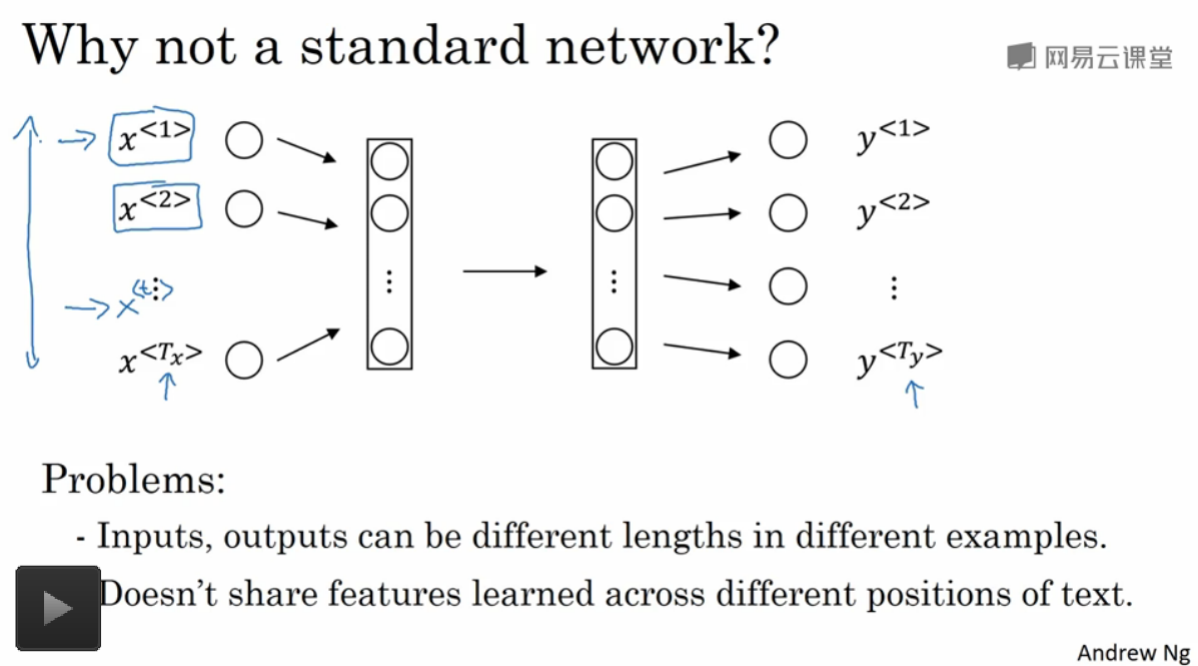## 2.RNN结构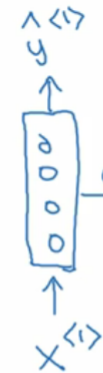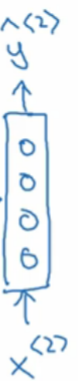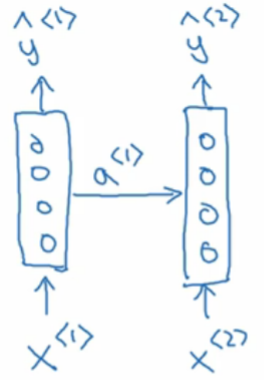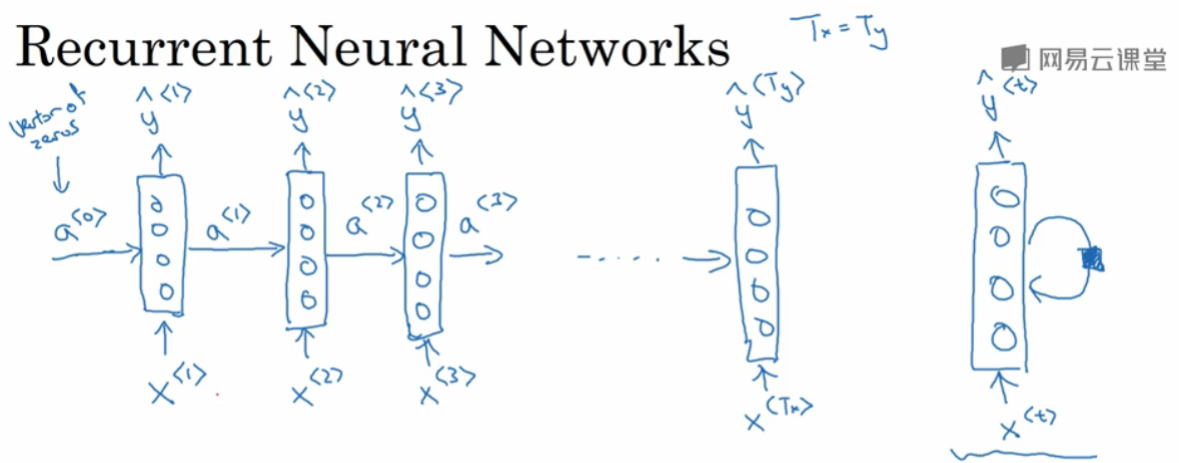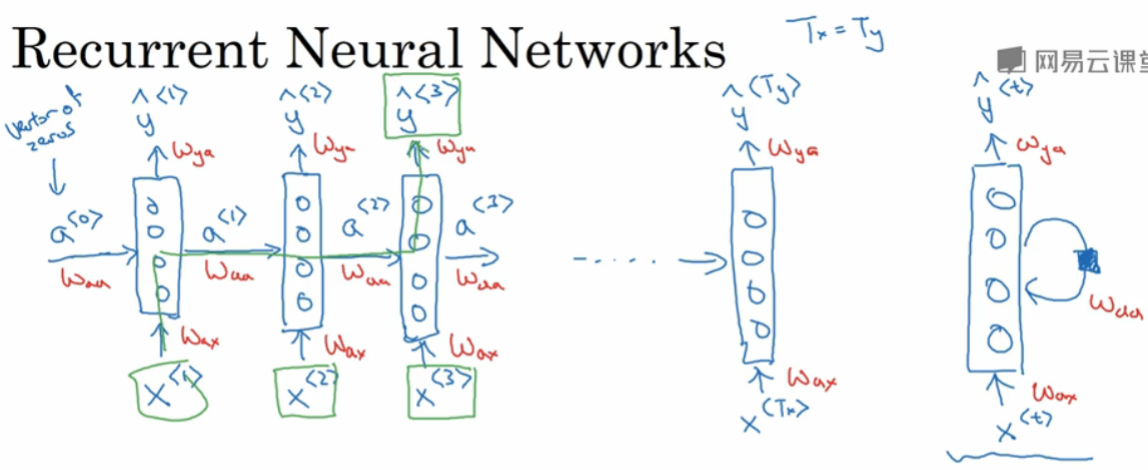## 3.RNN前向传播

• $$a^{<1>}=g_1(W_{aa}a^{<0>}+W_{ax}x^{<1>}+b_a)$$
• $$y^{<1>}=g_2(W_{ya}a^{<1>}+b_y)$$
• $$a^{<t>}=g_1(W_{aa}a^{<t-1>}+W_{ax}x^{<t>}+b_a)$$
• $$y^{<t>}=g_2(W_{ya}a^{<t>}+b_y)$$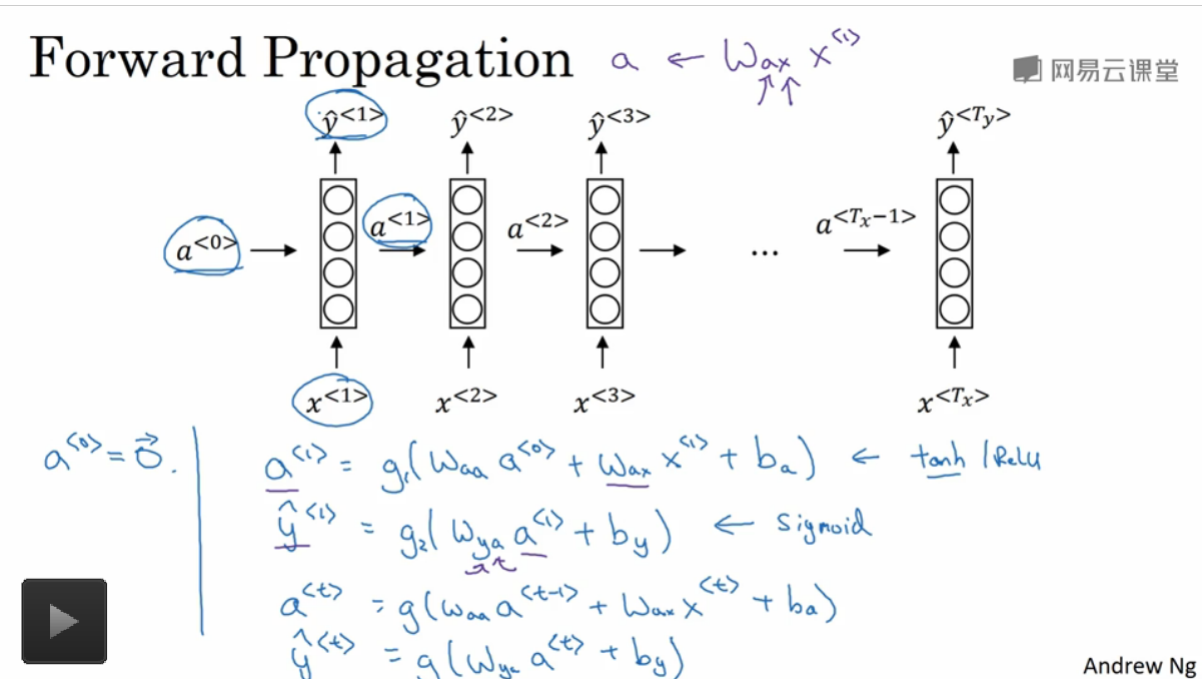## 4.简化RNN公式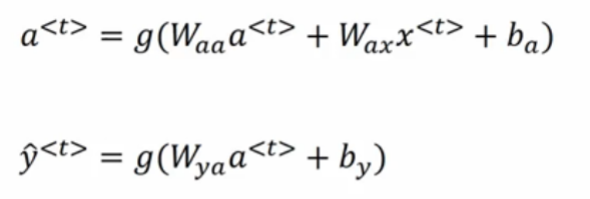• 1. 简化$$a^{<t>}$$

\begin{align} a^{<t>}&= g(W_{aa}a^{<t-1>}+W_{ax}x^{<t>}+b_a) \notag \\ &= g(W_a [a^{<t-1>},x^{<t>}]^{T}+b_a) \notag \end{align}• $$W_a = [ W_{aa}, W_{ax}]$$,假设$$W_{aa}$$是(100,100)的矩阵，$$W_{ax}$$是(100,10000)的矩阵,那么$$W$$则是(100,10100)的矩阵。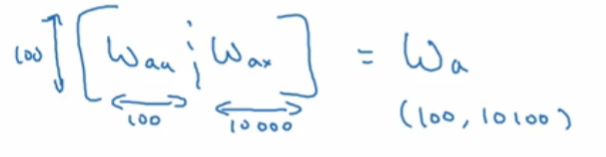• $$[a^{<t-1>},x^{<t>}]^T$$是下图示意。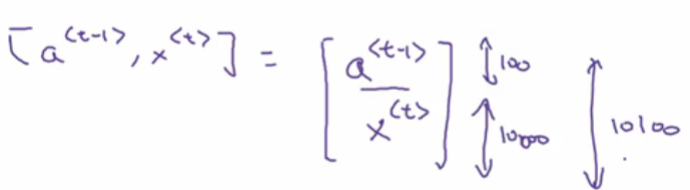$$W_a [a^{<t-1>},x^{<t>}]^{T}$$矩阵计算如下图示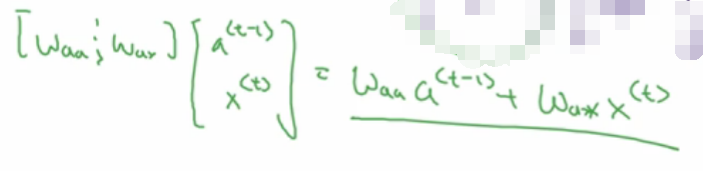• 2.简化$$y^{<t>}$$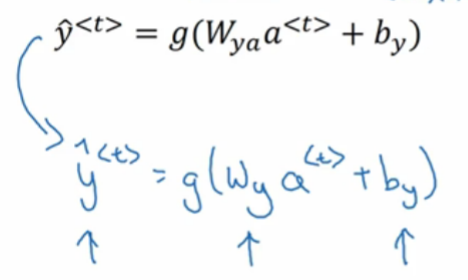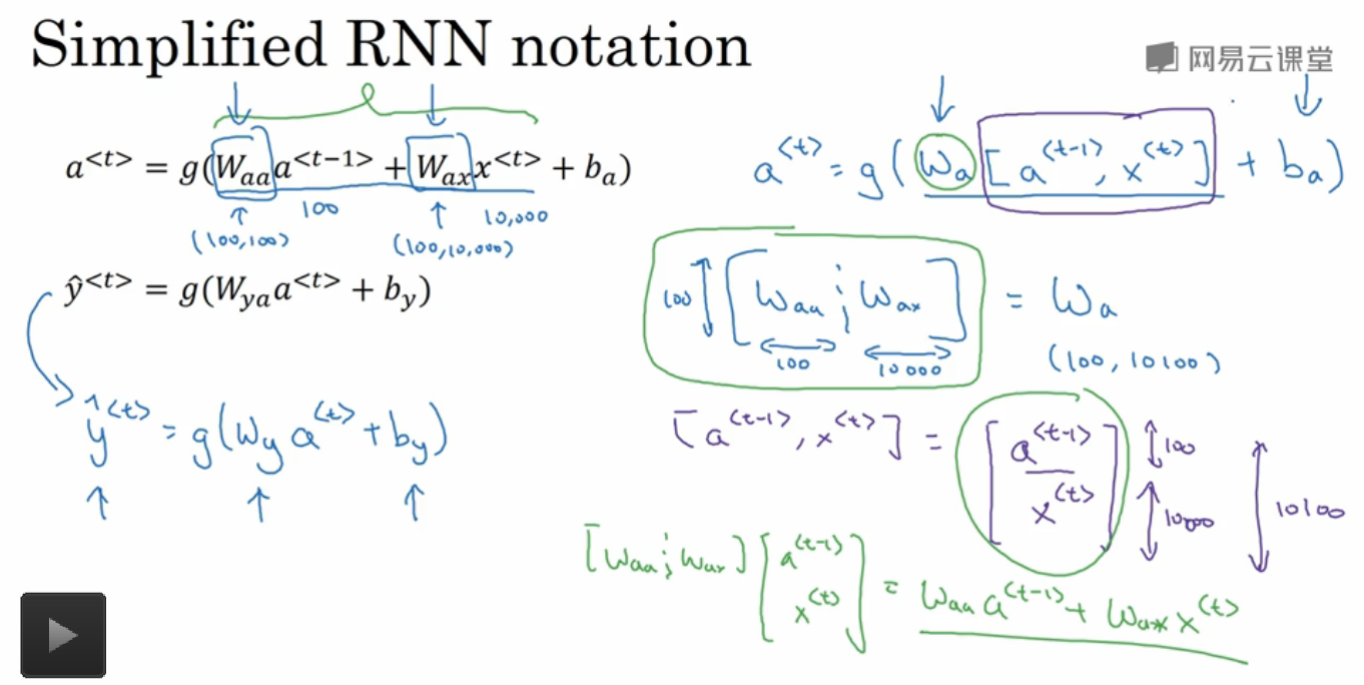# 四、通过时间的反向传播

## 1.整体感受

• 首先再回顾一下RNN的整体结构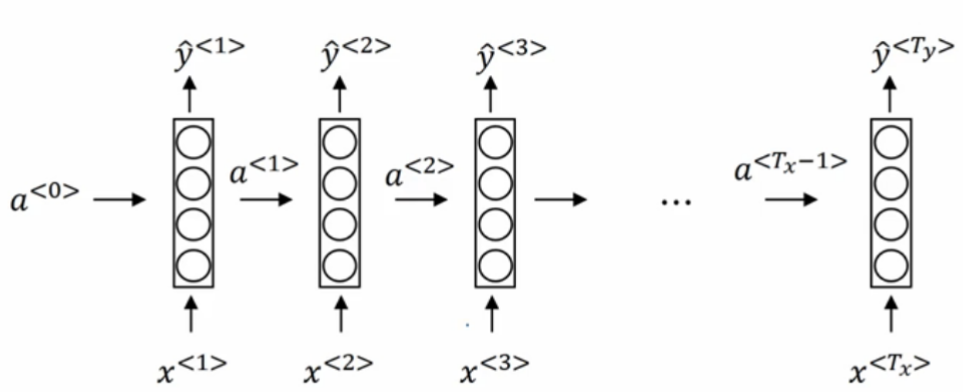• 要进行反向传播，首先需要前向传播，传播方向如蓝色箭头所示，其次再按照红色箭头进行反向传播。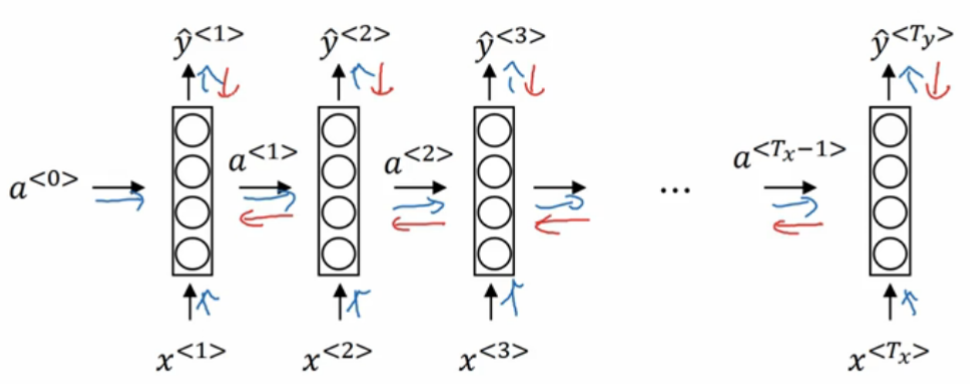## 2.前向传播

• 首先给出所有输入数据，即从$$x^{<1>}$$$$x^{<T_x>}$$,$$T_x$$表示输入数据的数量。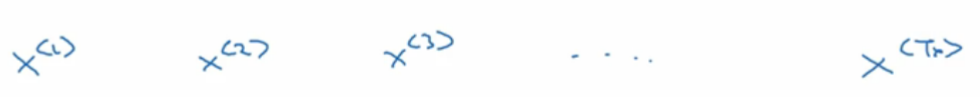• 初始化参数$$W_a,b_a$$，将输入数据输入网络得到对应的$$a^{<t>}$$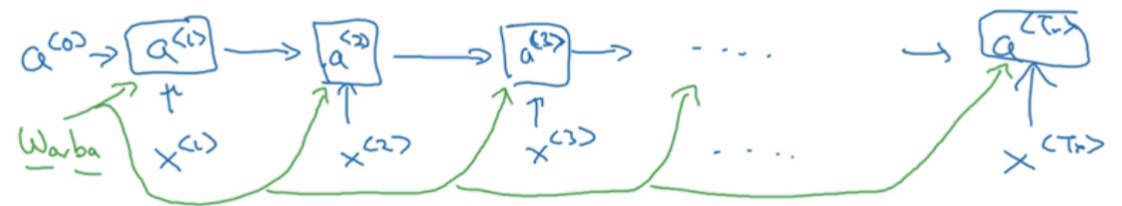• 再通过与初始化参数$$W_y,b_y$$得到$$y^{<t>}$$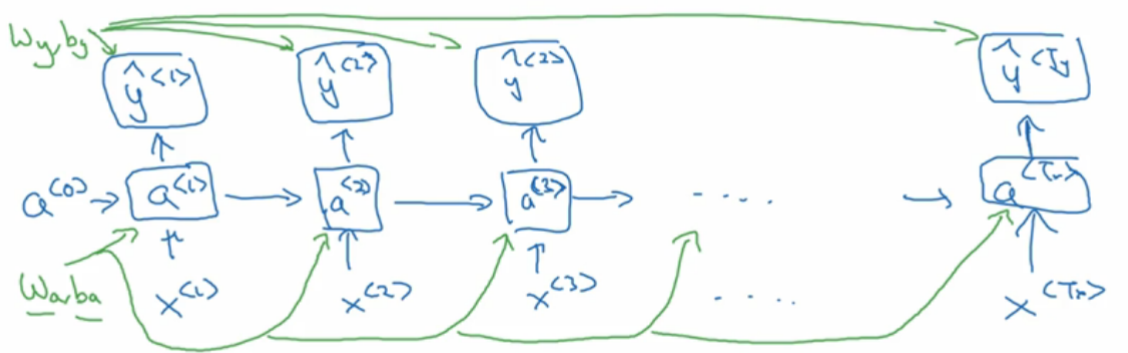## 3.损失函数定义

• 每个节点的损失函数

$$L^{<t>}(\hat{y}^{<t>},y^{<t>})=y^{<t>}log(y^{<t>})-(1-y^{<t>})log(1-\hat{y}^{<t>})$$

• 整个网络的损失函数

$$L(\hat{y}^{<t>},y^{<t>)}) = \sum_{t=1}^{T_y}L^{<t>}(\hat{y}^{<t>},y^{<t>})$$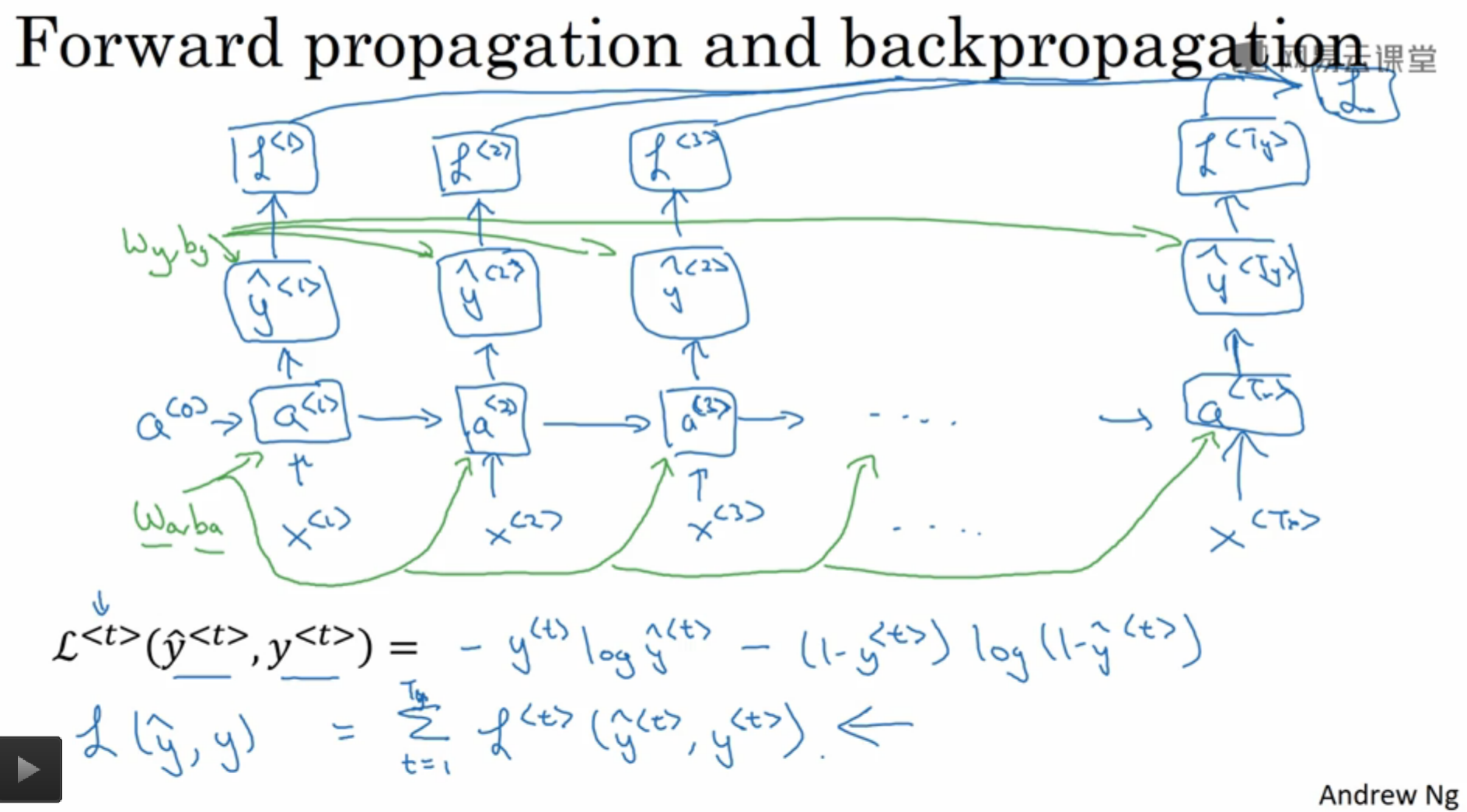## 4.反向传播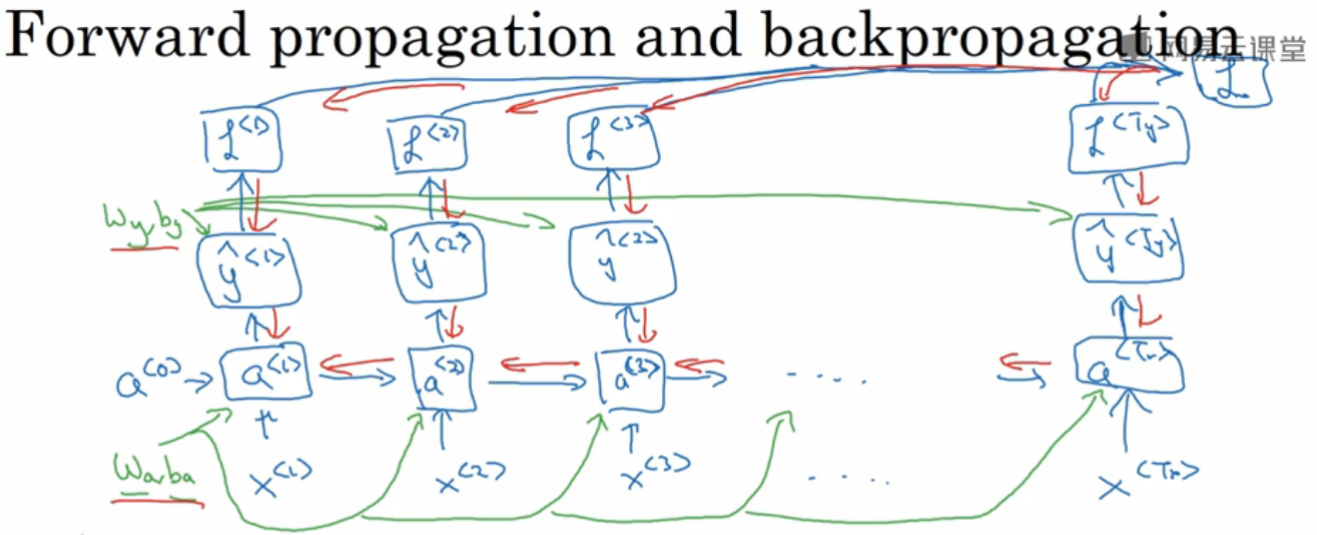## 5.整个流程图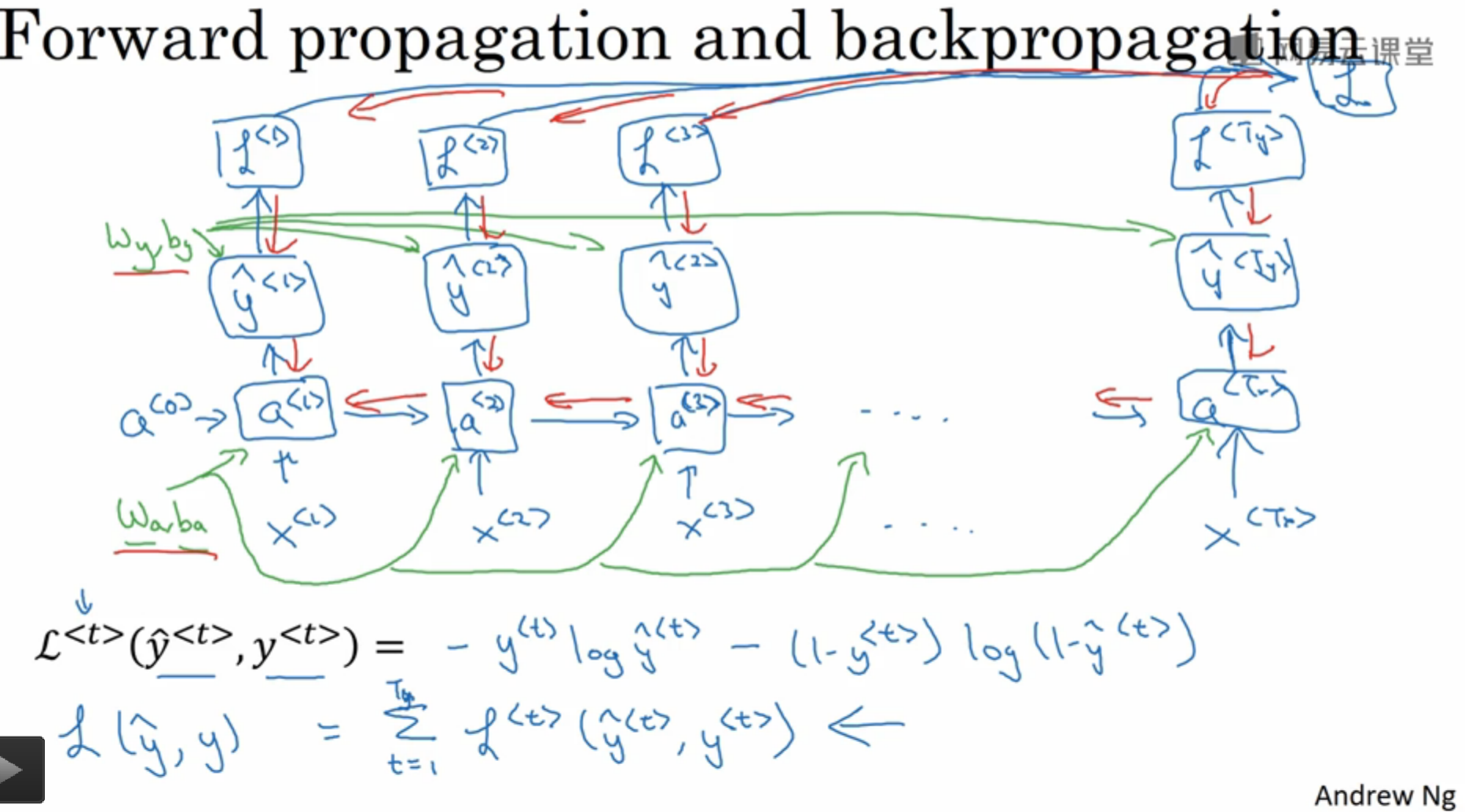# 五、不同类型的循环神经网络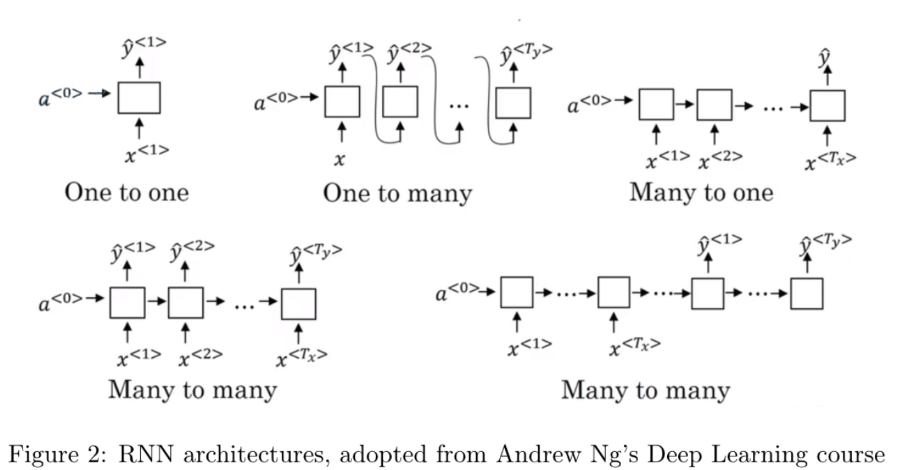# 六、语言模型和序列生成

## 1.什么是语言模型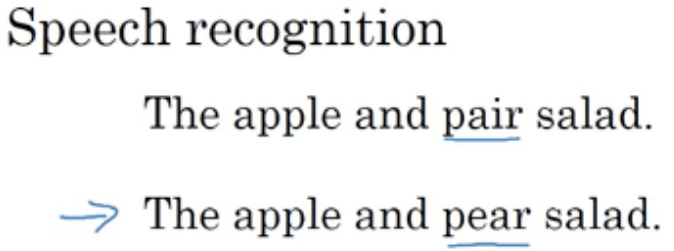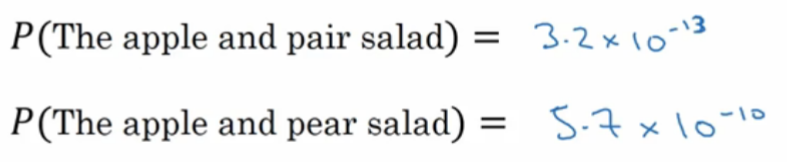## 2.如何使用RNN构建语言模型

• 首先我们需要一个很大的语料库(Corpus)
• 将每个单词字符化(Tokenize，即使用One-shot编码)得到词典,，假设有10000个单词
• 还需要添加两个特殊的单词
• : end of sentence. 终止符，表示句子结束.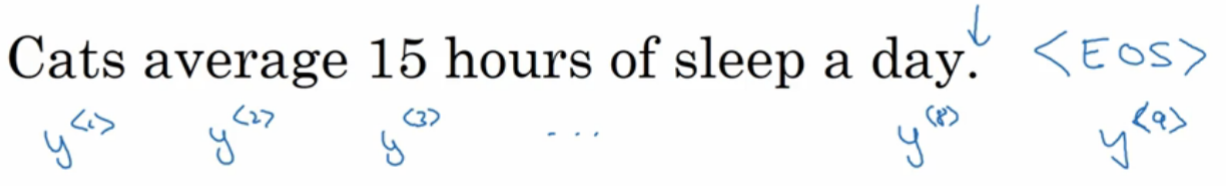• : UNknown, 之前的笔记已介绍过.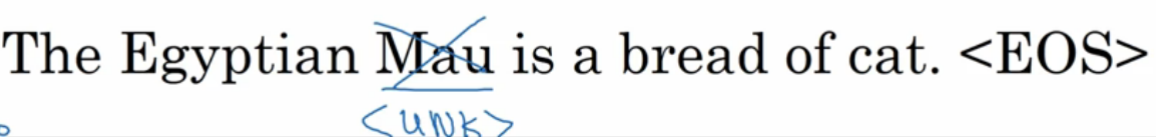## 3.构建语言模型示例

• 1.初始化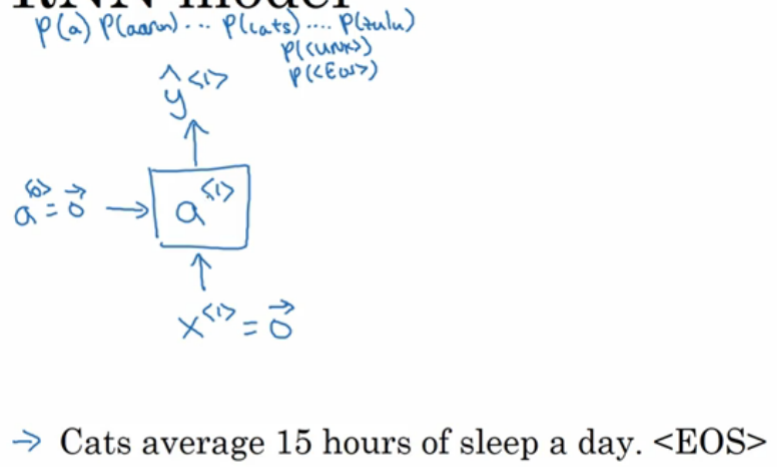• 2.将真实值作为输入值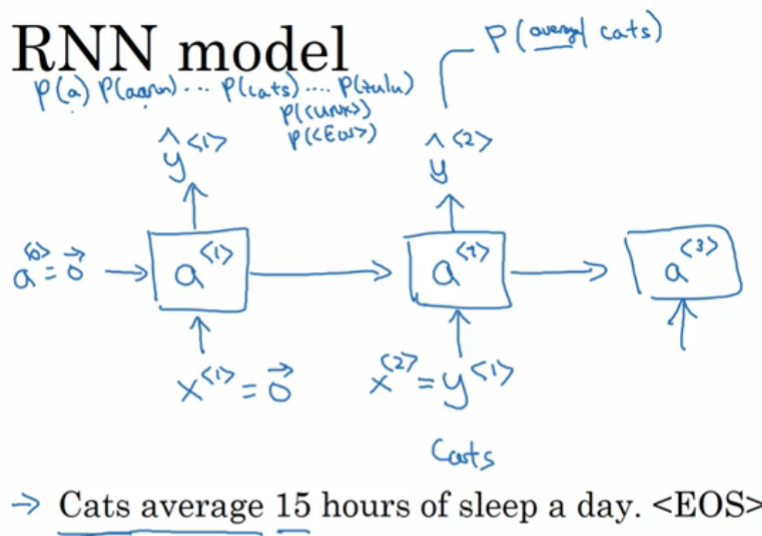• 3.计算出损失值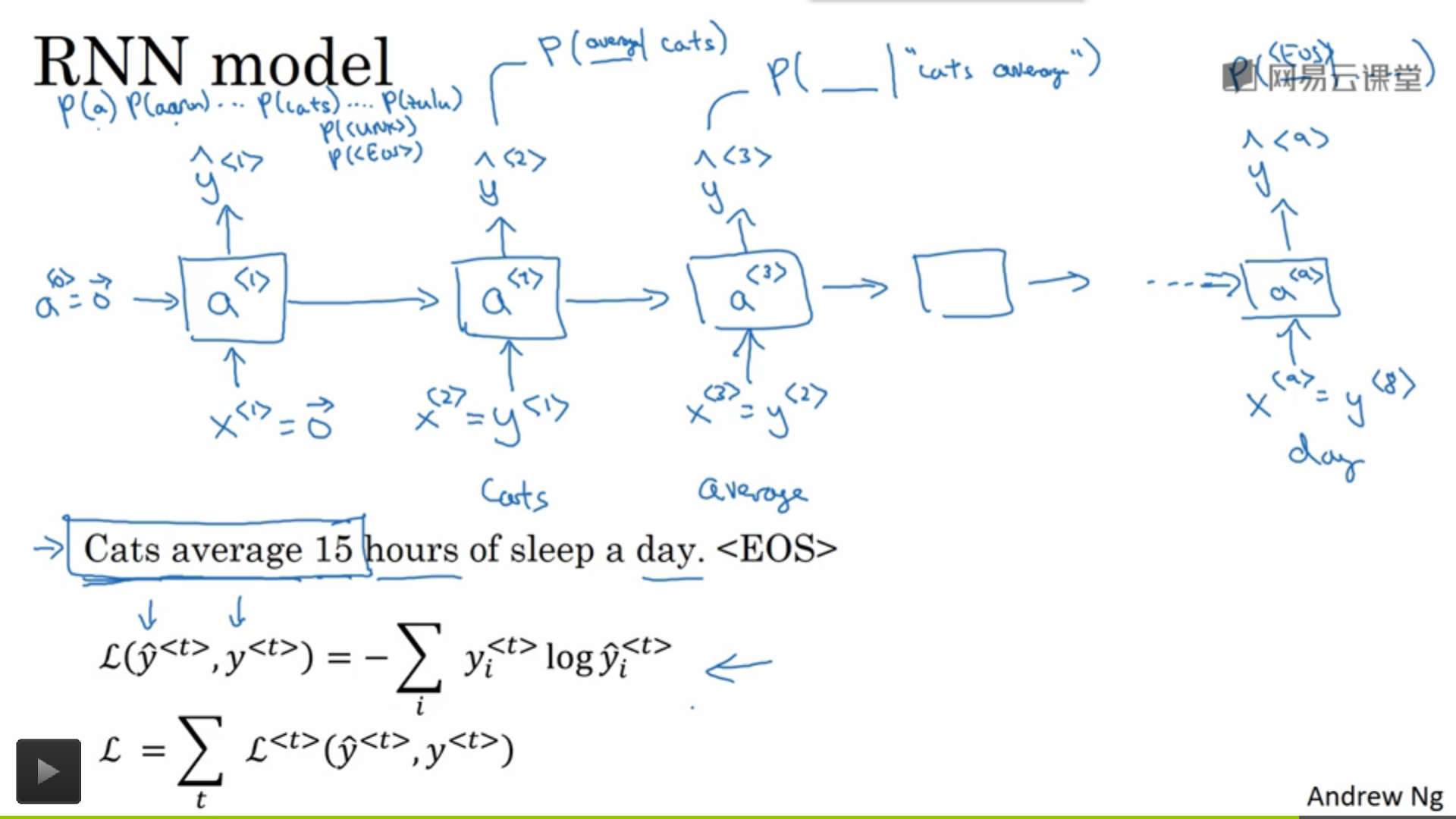# 七、对新序列采样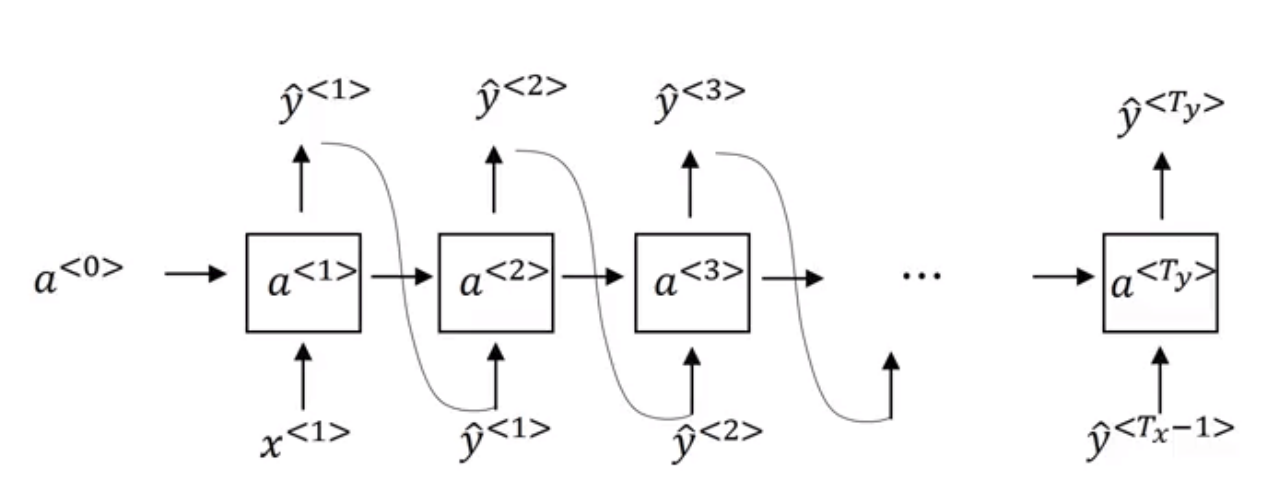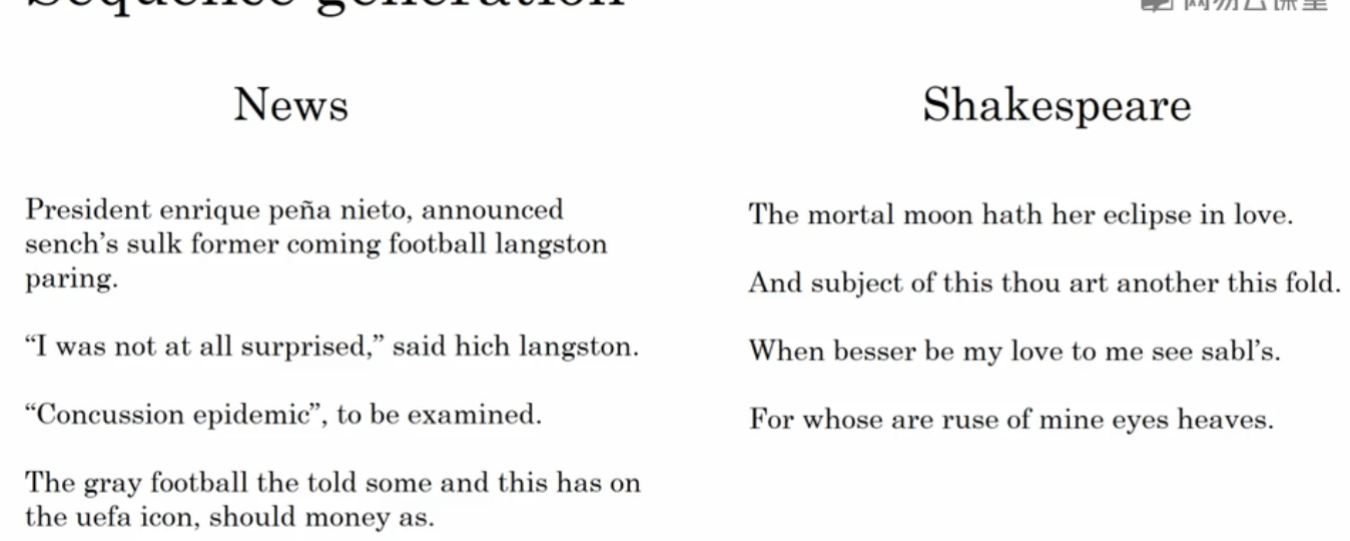# 八、带有神经网络的梯度消失

## 1.RNN的梯度消失、爆炸问题

• 当梯度值过小的时候，神经网络将无法有效地调整自己的权重矩阵导致训练效果不佳，称之为“梯度消失问题”(gradient vanishing problem)
• 过大时可能直接影响到程序的运作因为程序已经无法存储那么大的值，直接返回 NaN ，称之为“梯度爆炸问题”(gradient exploding problem)

if (gradient > max) {
}

“The cat，which already ate apple，yogurt，banana，..., was full.”

“The cats，which already ate apple，yogurt，banana，..., were full.”

# 九、GRU单元

GRU（Gated Recurrent Unit）是一种用来解决梯度值过小的方法，首先来看下在一个时刻下的RNN单元，激活函数为 tanh

## 1.首先回顾一下普通RNN单元的结构示意图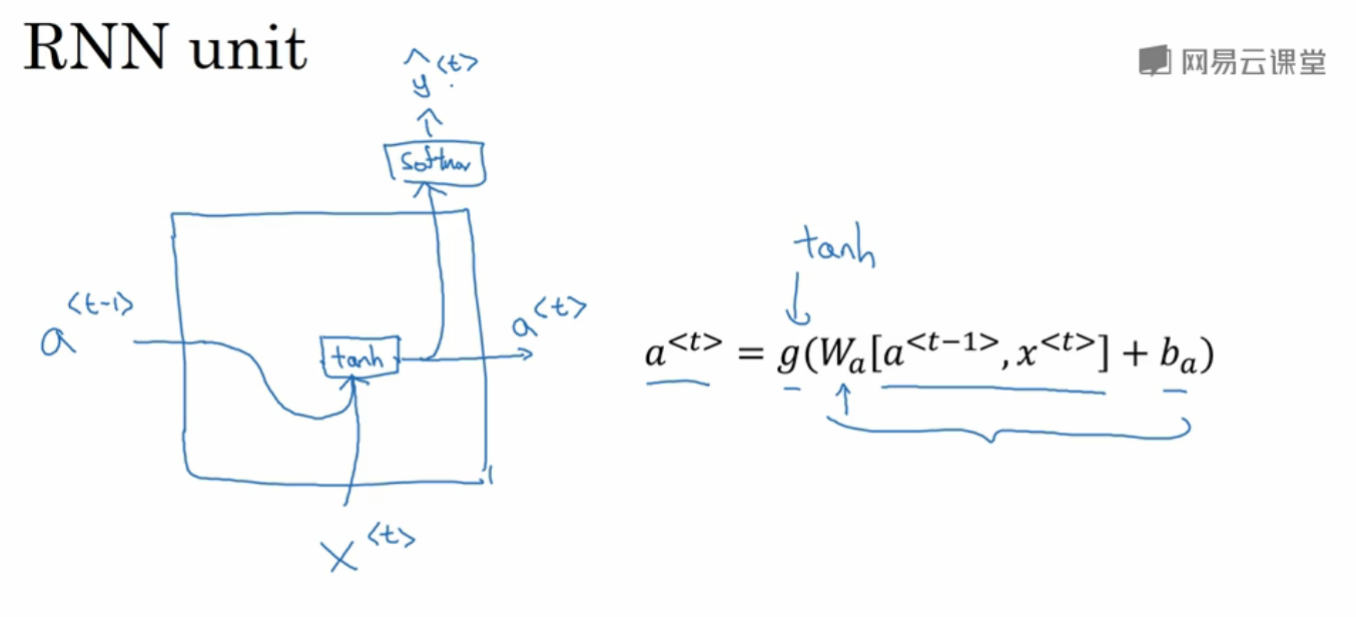## 2.GRU结构

• 记忆细胞

$\tilde{c}=tanh(W_c [c^{<t-1>}, x^{<t>}]+b_c)$

• 更新门(update gate)

$\Gamma_u=σ(W_u [c^{<t-1>}, x^{<t>}]+b_u)$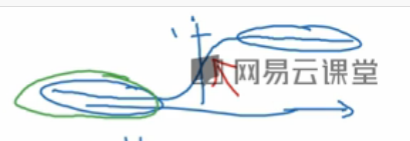• t时刻记忆细胞

$c^{<t>}=\Gamma_u*\tilde{c}+(1-\Gamma_u)*c^{<t-1>}$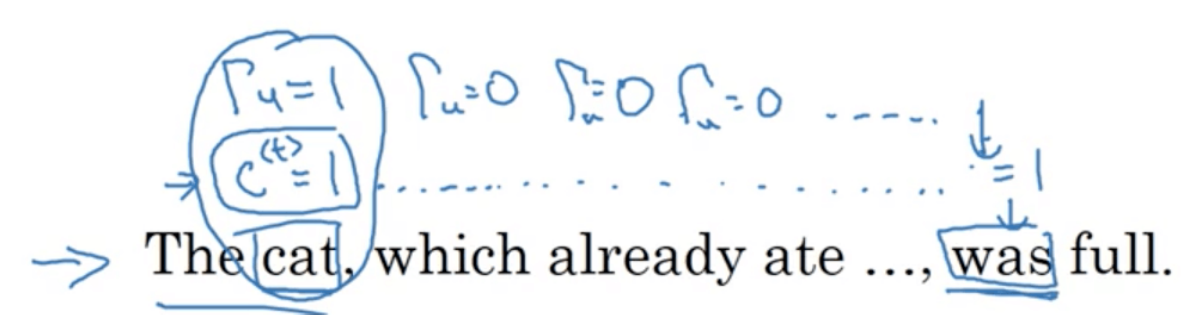• GRU结构示意图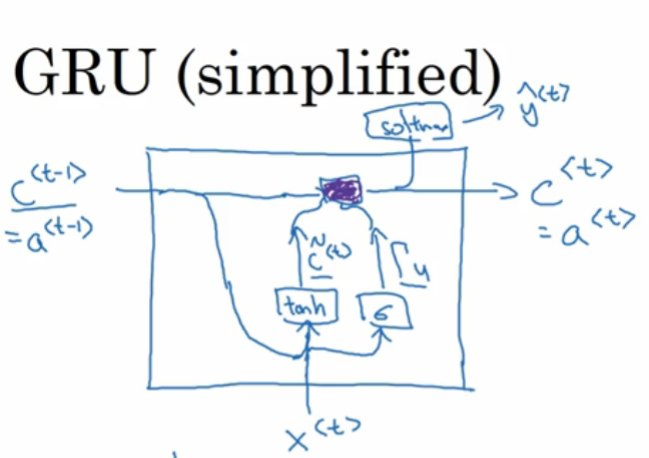## 3.完整版GRU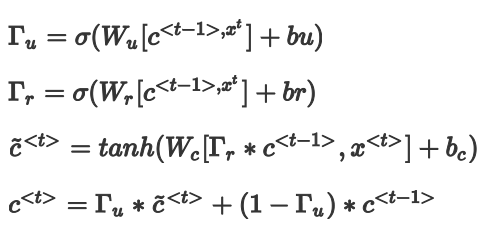# 十、长短期记忆

GRU只有两个门，而LSTM有三个门，分别是更新门$$\Gamma_u$$(是否需要更新为$$\tilde{c}^{<t>}$$)，遗忘门$$\Gamma_f$$(是否需要丢弃上一个时刻的值)，输出门$$\Gamma_o$$(是否需要输出本时刻的值)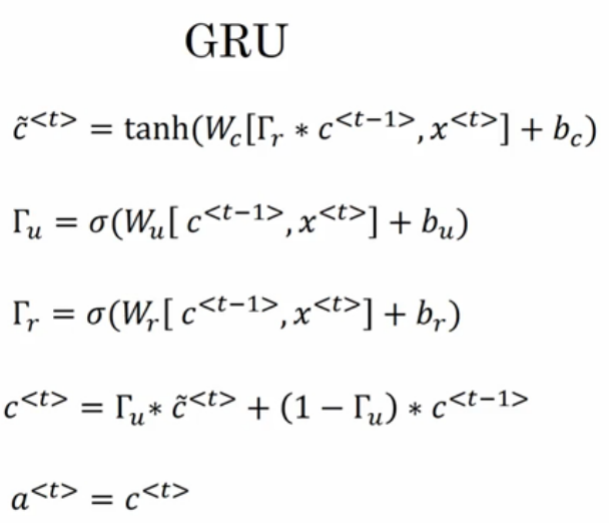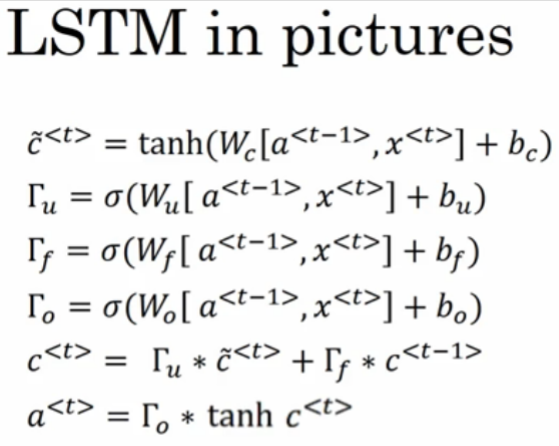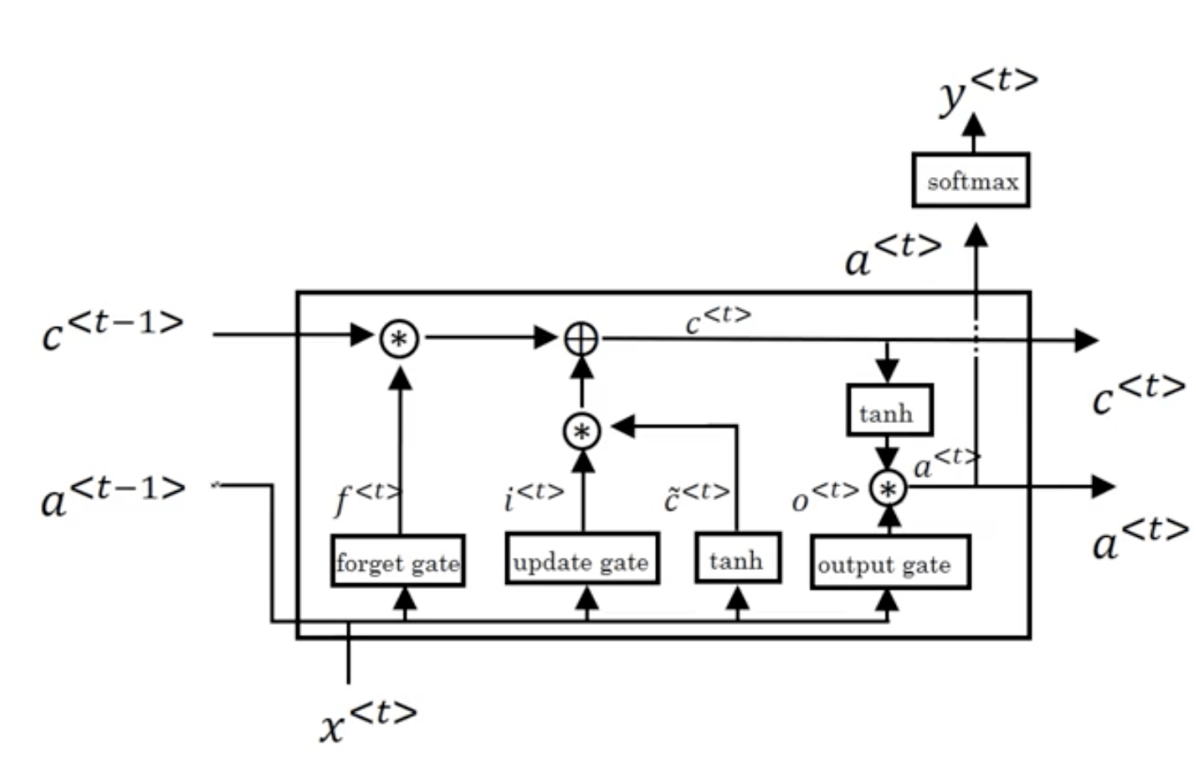# 十一、双向递归神经网络

He said, "Teddy Roosevelt was a great President".
He said, "Teddy bears are on sale".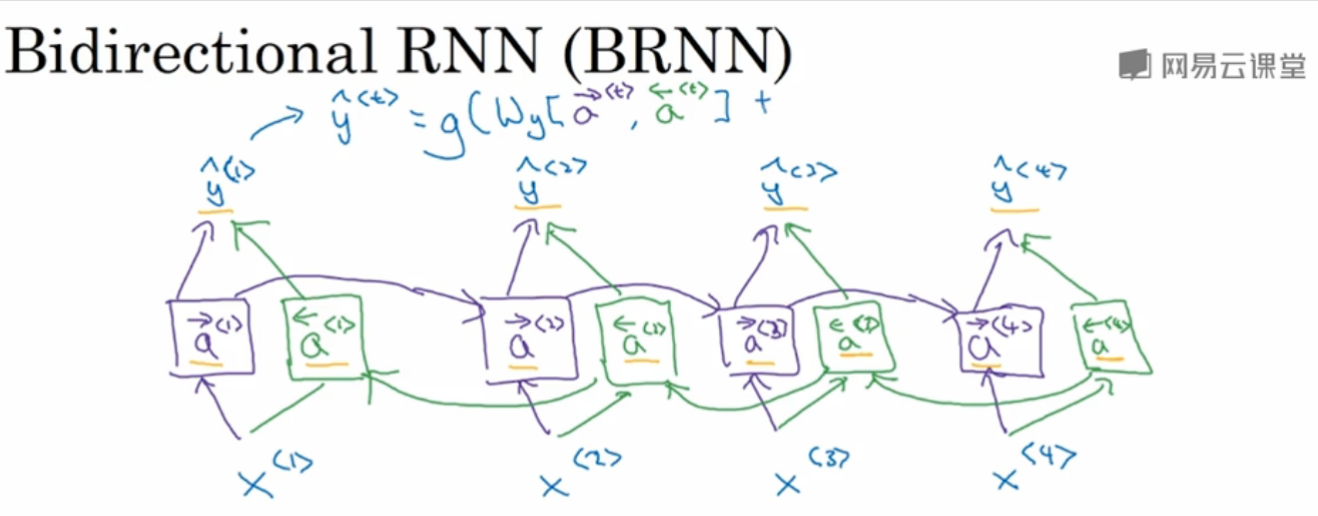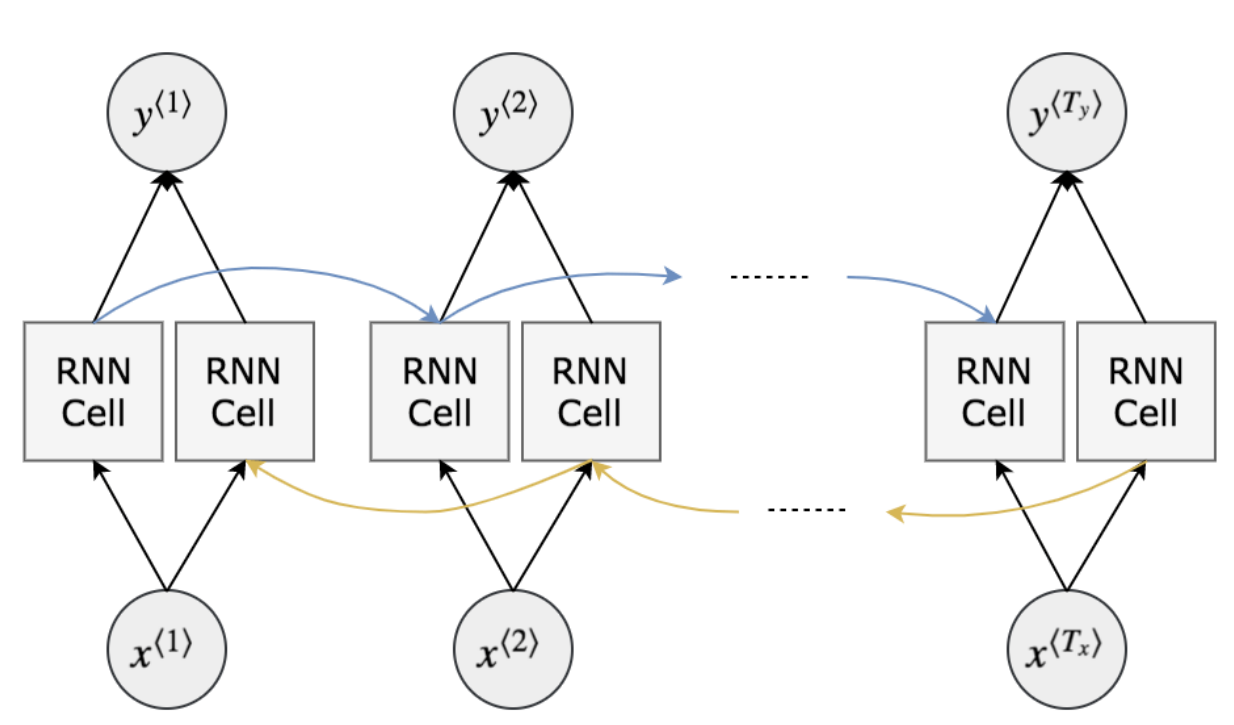# 十二、深层循环神经网络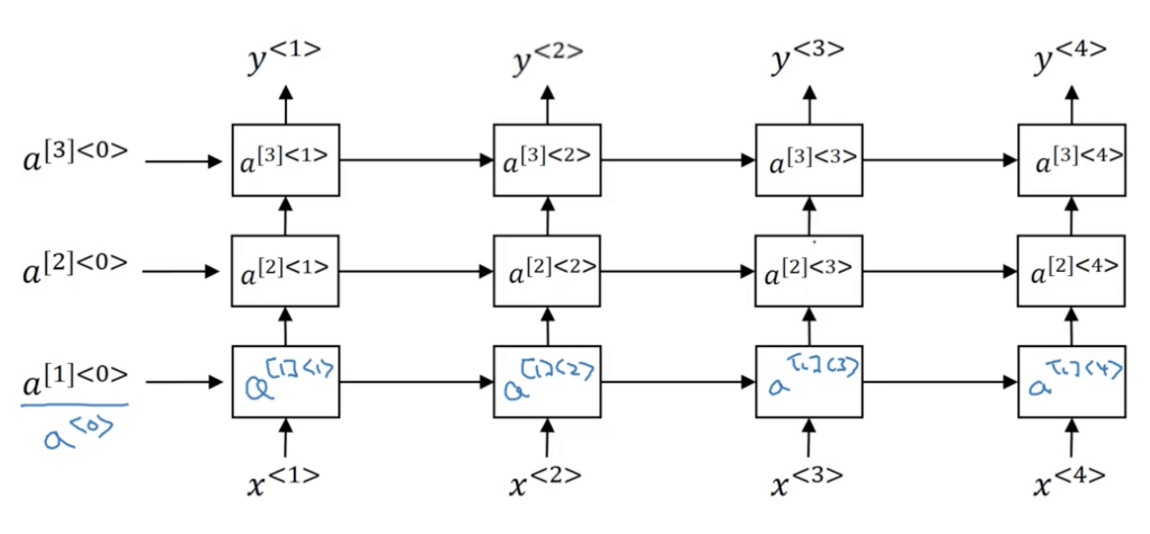• 表示第一层
• <0>表示第一个激活值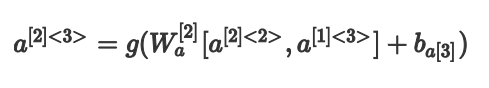### MARSGGBO原创 2018-1-17do-while 循环|学习笔记

48 0AI学习笔记——Q Learning

1059 0title: 斯坦福-随机图模型-week4.0 tags: note notebook: 6- 英文课程-9-Probabilistic Graphical Models 1: Representation --- 斯坦福-随机图模型-week4.0 最大期望收入模型 简答的决策 我们使用随机图模型进行决策需要的原料是什么ne ? 我们需要决策的情景 一些列的可能的行为 一系列的转台量： 还有一个收益函数 期望的收益公式： 期望收益公式表示是这样的，每个行为的可能性，乘以他的期望收益的加权和。
674 0title: 斯坦福-随机图模型-week2.0 tags: note notebook: 6- 英文课程-9-Probabilistic Graphical Models 1: Representation --- 斯坦福-随机图模型-week2.0 模板模型（Template Models） 在实际的模型的建立的过程中，会出现很多的重复的情况。
837 0title: 斯坦福-随机图模型-week1.5 tags: note notebook: 6- 英文课程-9-Probabilistic Graphical Models 1: Representation --- 斯坦福-随机图模型-week1.5 贝叶斯网络 朴素贝叶斯 朴素贝叶斯是一个概率的分类模型，下面我们用概率图的思想去理解他。
671 0WebSocket 学习笔记--IE，IOS，Android等设备的兼容性问题与代码实现
1820 05289 031185 0
marsggbo
AutoML
159

0